# Introduction

Memory Evolutive Systems:
Categorical modelisation of hierarchical, evolving and self-regulating systems (≃ 30 years of research already!).

### Authors

A. EhresmannMathematician (Université de Picardie):
1. Functional analysis
2. Category theory with Charles Ehresmann
3. Transdisciplinary research: natural complex systems (biological, social, cognitive)
J.-P. Vanbremeersch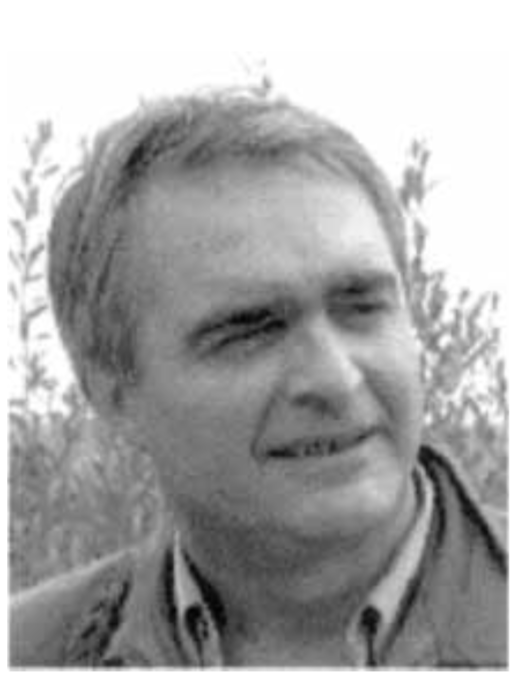Physician (Université de Picardie): specialty in geriatry

# What’s wrong with biology?

## 1. Emergence vs. Reductionism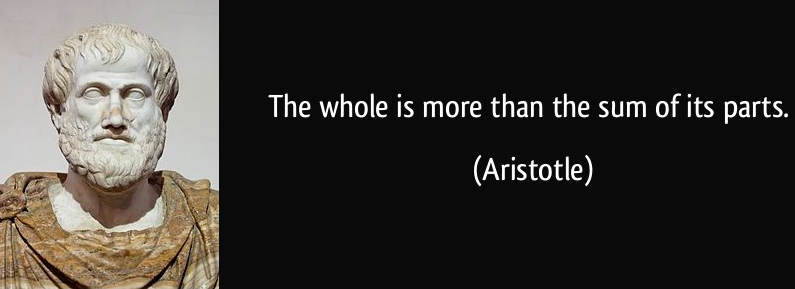## 2. Self-organisation

• Flexibility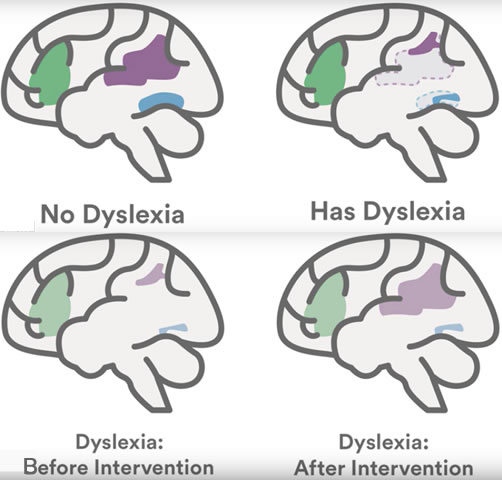Dyslexia - Key parts of the brain not (→ but may become) developed for reading

# I. Make room for… Category theory!

Structures preserved by morphisms$\qquad \overset{\text{+ associativity, identity}}{\rightsquigarrow} \qquad \underbrace{\text{Category Theory}}_{\text{focus on relations rather than objects}}$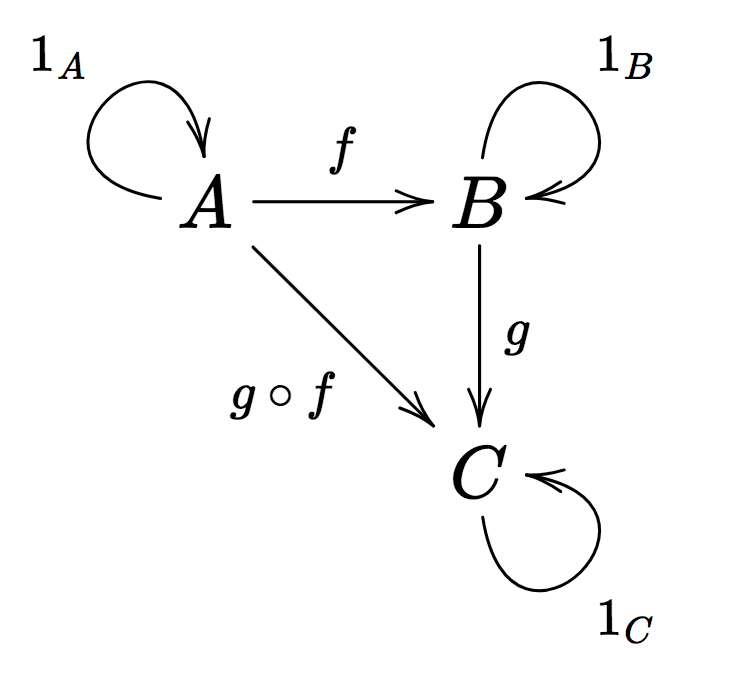Examples:

• Objects preserved by morphisms:

• Sets
• Vector spaces, Topological spaces, Manifolds, Groups, Rings, …
• Logical formulas, Types (λ-calculus), …
• Structures forming one category:

• Posets, Monoids, Groups, Groupoids, …

## … and even (small) categories themselves form a category!

• Objects: Categories
• Morphisms: Functors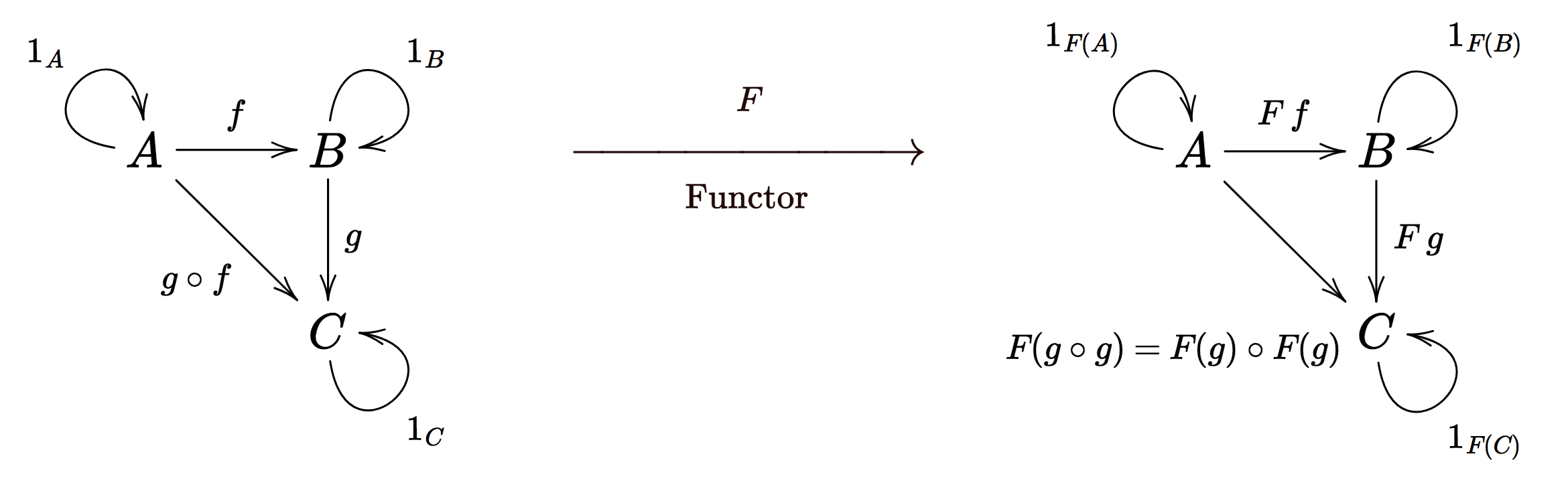## Universal constructions

Limits:
• Terminal objects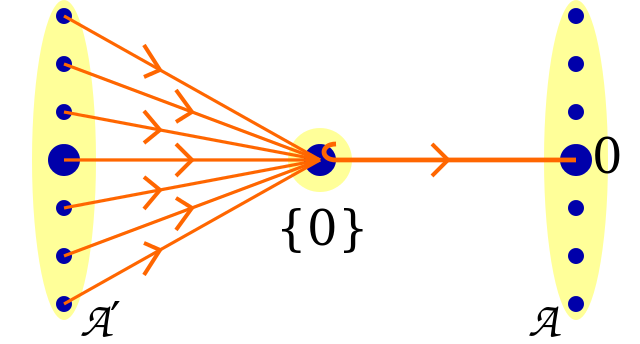• Products• Equalizers (Kernels), Pullbacks, …

Colimits:
• Initial objects

• Coproducts• Coequalizers (Quotients), Pushouts, …

⟹ Colimits are more than sums

### Complex objects as colimits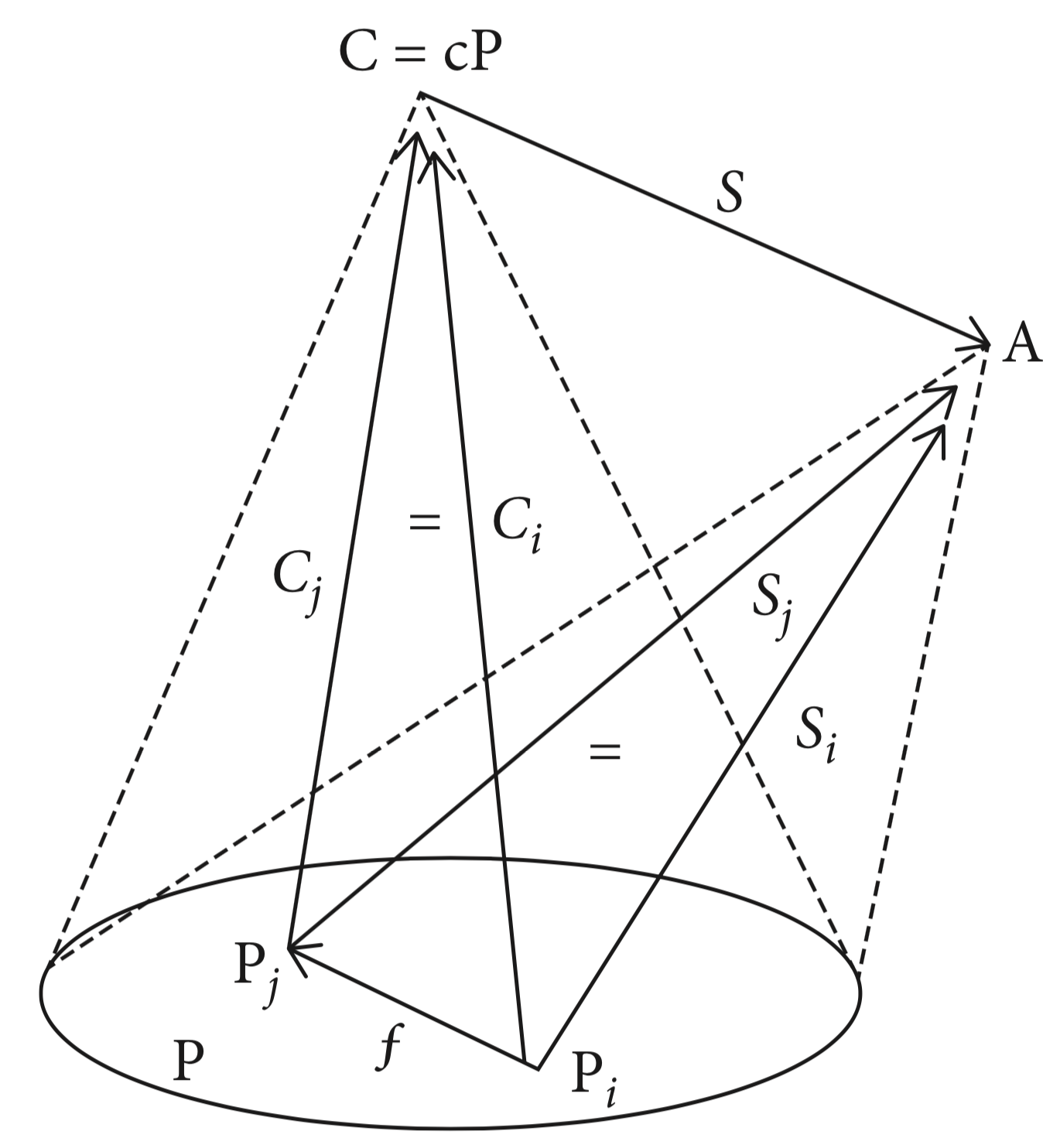Figure - Colimit of a pattern/diagram

Hierarchical category $H_t$: layers of complexity: objects at level $n$ are colimits of patterns of level $<n$

# II. Hierarchical Evolutive Systems (HES)

Hierarchical Evolutive System (HES) $K$:
• a timescale $T ⊆ ℝ_+$
• $∀ t∈ℝ_+$, a hierarchical category $K_t$ (configuration at $t$)
• $∀ t < t'$, a transition functor $k_{tt'}: K_{tt'} ⊆ K_{t} ⟶ K_{t'}$
• Transitivity condition: a component $C ∈ H$ is a maximal set of objects linked by transitions.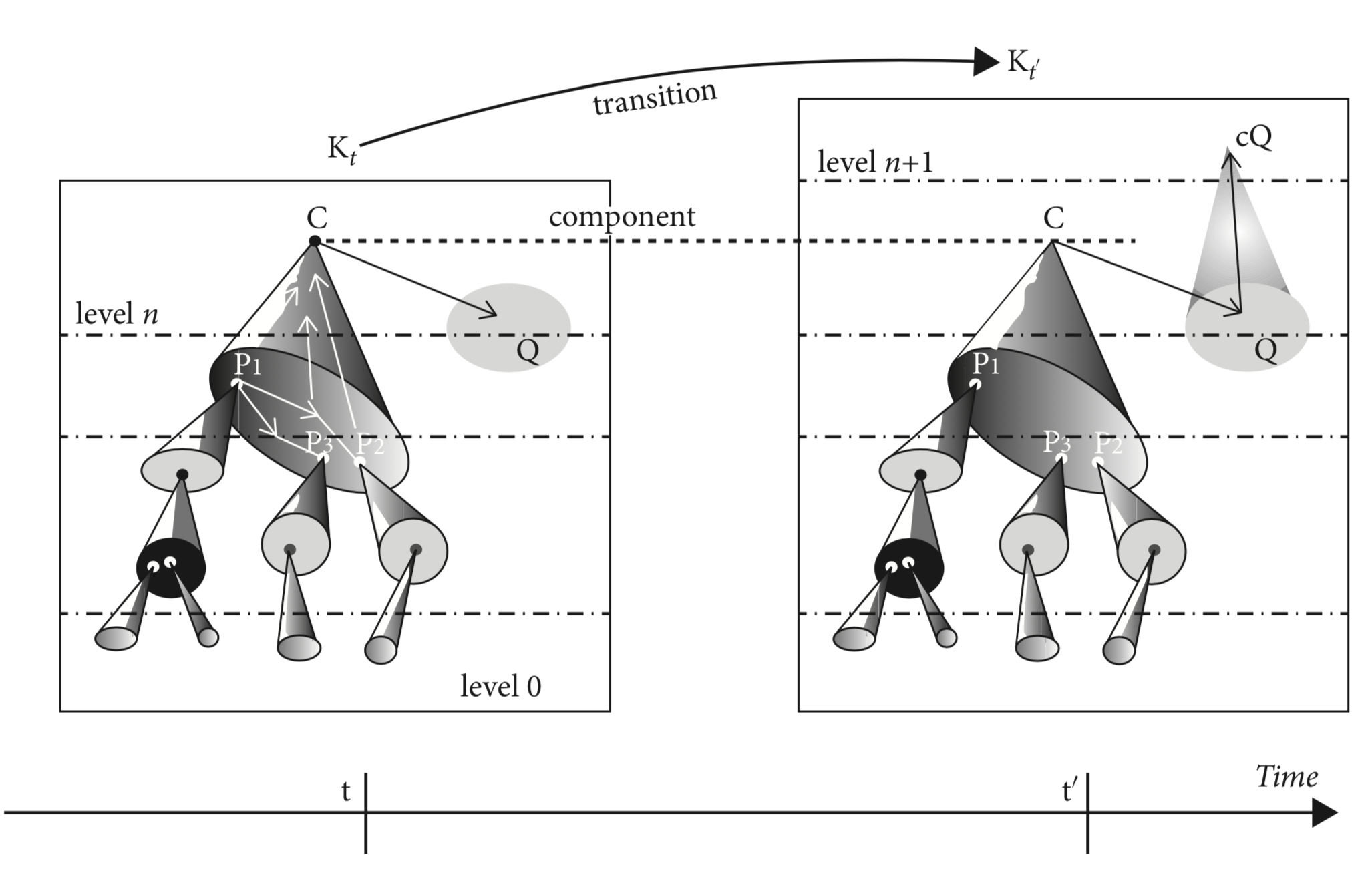Figure - a Hierarchical Evolutive System (HES) $K$

# 3. Multiplicity Principle

Multiplicity Principle: for each time and each level, there is at least one multi-faceted component, i.e. two patterns with the same colimit that are not isomorphic in the category of patterns and clusters.

# Complexification

“Standard changes” of living systems (cf. René Thom): birth: (addition of new components), death (addition of certain components), collision (formation of new pattern bindings), scission (destruction of certain bindings).

## Emergence Theorem

Complexification preserves the Multiplicity Principle.

In a HES, there may be:

• formation of multi-faceted components more and more complex
• ⟶ emergence of complex links between them.

## III. Memory Evolutive Neural Systems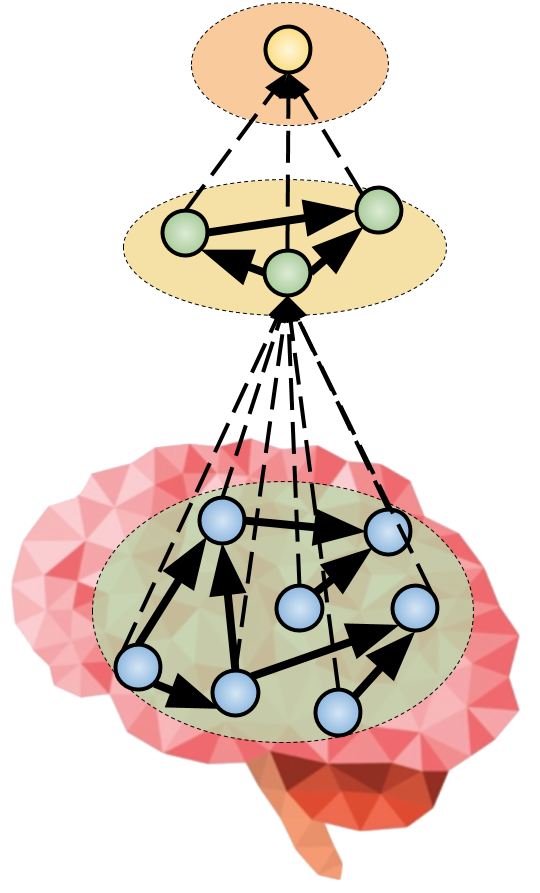## Flexible Memory

### vs. Hopfield networks

Hopfield rule:

to store patterns $\textbf{p}_1, ⋯, \textbf{p}_n$ in the Hopfield network, the weight matrix is set to:

$$W = \frac 1 N \sum\limits_{ i } \textbf{p}_i \textbf{p}_i^\T$$

cf. Jupyter Notebook for simulations

Hopfield networks:

• the stored patterns are not apparent in the network
• pattern capacity: $≃ 0.138 N$ (where $N$ is the number of neurons)
• no “flexibility”: if your mom’s appearance changes when she ages (which is unfortunately likely to happen), you don’t recognize her anymore!
• spurious patterns: linear combinations of odd number of patterns also stored!
• unoriented graph!